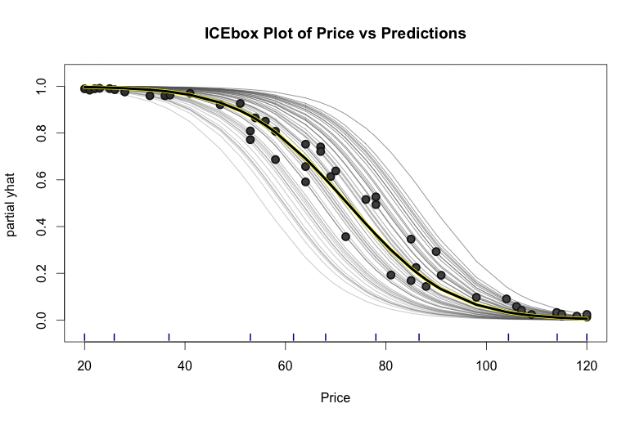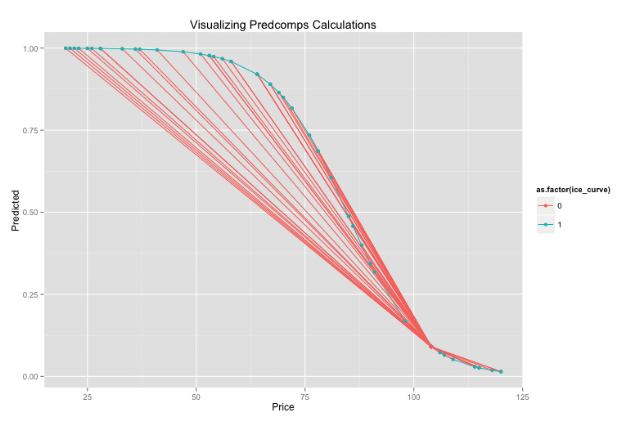## Sunday, December 6, 2015

### Beyond Beta: Relationships between partialPlot(), ICEbox(), and predcomps()

Machine Learning models generally outperform standard regression models in terms of predictive performance. However, these models tend to be poor in explaining how they achieve a particular result. This post will discuss three methods used to peak inside "black box" models and the connections between them.

Ultimately the methods discussed here adhere to a similar principle: change the input and see out the output changes.

Suppose we have a model y=f(u,v) and we're interested in the effect of u on the output of the function keeping v constant. These methods change u while keeping other variables, v, constant. For a particular observation x_i; predict with all unique values of u while keeping x_i constant.

for q in  (unique values in u);
fhat(q)=predict(q,v)

Now we have a pair of data of what the predicted value of y over the set of unique values of u keeping everything else constant.

Below is a plot that shows what happens when we plot these predicted values, changing u while keeping other variables, v, constant.  (The code was taken from from David Chudzicki's Blog Post). It is a logistic regression with two variables. (Example was chosen to demonstrate the connections between the functions described here. One generally wouldn't use these techniques with a logistic regression. These are more appropriate for complex ensemble or hierarchal models.) The plot shows what the prediction is if we kept one variable constant while the other variable changed. In this case the variable Price changes while keeping everything else constant.If we repeat this procedure for every single observation, we can see what ICEbox package does. Below are two plots; 1) ICE plots using predcomps code calculations and ggplot 2) ICE plots using ice() function:The above plots show that the two functions have similar calculations.

partialPlot() in randomforest takes the mean at a particular unique value. partialPlot is basically an aggregated value of ICEbox. In this case the line in ICEbox chart (bold and colored) is the equivalent to partialPlot() results. There are many cases where interaction effects are masked by taking the average effect, so visualizing ICE curves is more informative than partiaPlot().

The goal of predcomps() isn't necessarily to visualize how variables are related, it's to find something similar to a "beta" coefficient for complex models. That is, it takes the average change in prediction / change in variable of interest (u). Following the example from the first plot, this means its the average slope of all predicted, unique data points relative to the original data point. The resulting graph is shown below.Above we see the original data point has a price of 104. It calculates the weighted average (the weight is the mahalanobis distance between the points) slope in regards to this point (lines shown as factor 0). To obtain the overall predcomps "beta" it does this for all observations (or as many as selected by the user).

This post started out predicted n samples from one observation to create a single observation plot over range of interest u. Over many observations this gives us a ICE plots. aggregating together gives us a partialplot, and finding the (weighted) slope in regards to original data gives us the predcomps beta.

Code

Resources:
predcomps website
Average Predictive Comparisons Paper
Peeking Inside the Black Box: VisualizingStatistical Learning with Plots of IndividualConditional Expectation